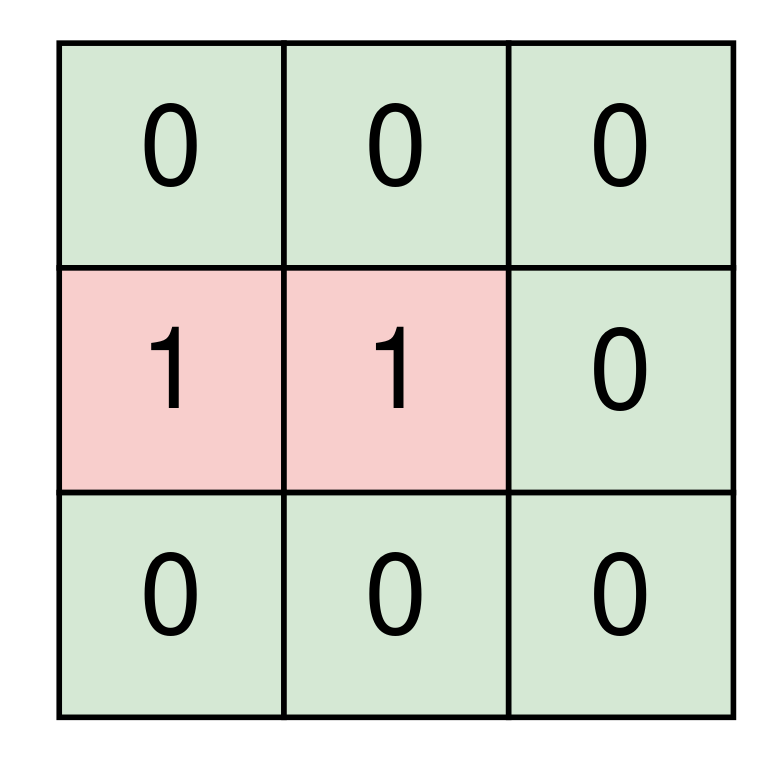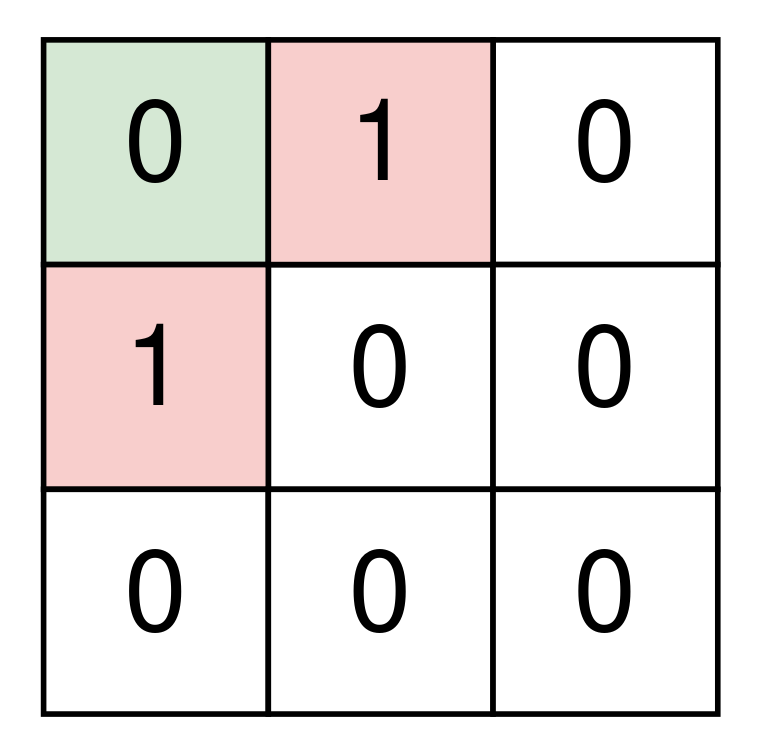### Standard Finite-State-Machine (FSM) Implementation

This problem exemplifies a standard implementation of a Finite State Machine (FSM), non-recursively. It simply follows the instructions of the robot until it finds a visited state. The only caveat is that a visited state is not only a given cell, but rather a given cell and the direction. Some basic math to create a key that identifies this state. The standard FSM then becomes: check for end condition, process, based on current state, either move or change state. Code is down below, cheers, ACC.

2061. Number of Spaces Cleaning Robot Cleaned
Medium

A room is represented by a 0-indexed 2D binary matrix `room` where a `0` represents an empty space and a `1` represents a space with an object. The top left corner of the room will be empty in all test cases.

A cleaning robot starts at the top left corner of the room and is facing right. The robot will continue heading straight until it reaches the edge of the room or it hits an object, after which it will turn 90 degrees clockwise and repeat this process. The starting space and all spaces that the robot visits are cleaned by it.

Return the number of clean spaces in the room if the robot runs indefinetely.

Example 1:```Input: room = [[0,0,0],[1,1,0],[0,0,0]]
Output: 7
Explanation:
The robot cleans the spaces at (0, 0), (0, 1), and (0, 2).
The robot is at the edge of the room, so it turns 90 degrees clockwise and now faces down.
The robot cleans the spaces at (1, 2), and (2, 2).
The robot is at the edge of the room, so it turns 90 degrees clockwise and now faces left.
The robot cleans the spaces at (2, 1), and (2, 0).
The robot has cleaned all 7 empty spaces, so return 7.
```

Example 2:```Input: room = [[0,1,0],[1,0,0],[0,0,0]]
Output: 1
Explanation:
The robot cleans the space at (0, 0).
The robot hits an object, so it turns 90 degrees clockwise and now faces down.
The robot hits an object, so it turns 90 degrees clockwise and now faces left.
The robot is at the edge of the room, so it turns 90 degrees clockwise and now faces up.
The robot is at the edge of the room, so it turns 90 degrees clockwise and now faces right.
The robot is back at its starting position.
The robot has cleaned 1 space, so return 1.
```

Constraints:

• `m == room.length`
• `n == room[r].length`
• `1 <= m, n <= 300`
• `room[r][c]` is either `0` or `1`.
• `room == 0`
Accepted
225
Submissions
351

```public int NumberOfCleanRooms(int[][] room)
{
Hashtable visitedSpaceAndDirection = new Hashtable();
int retVal = 0;

int row = 0;
int col = 0;
int direction = 1; //0:up, 1:right, 2:down, 3:left

int MULTIPLIER = 300 * 300 + 100;

for (; ; )
{
int key = direction * MULTIPLIER + row * room.Length + col;

if (visitedSpaceAndDirection.ContainsKey(key))
{
break;
}

if (room[row][col] == 0) retVal++;
room[row][col] = 2;

//Move
switch (direction)
{
case 0:
if (row > 0 && room[row - 1][col] != 1)
{
row--;
}
else
{
direction = (direction + 1) % 4;
}
break;
case 1:
if (col < room[row].Length - 1 && room[row][col + 1] != 1)
{
col++;
}
else
{
direction = (direction + 1) % 4;
}
break;
case 2:
if (row < room.Length - 1 && room[row + 1][col] != 1)
{
row++;
}
else
{
direction = (direction + 1) % 4;
}
break;
case 3:
if (col > 0 && room[row][col - 1] != 1)
{
col--;
}
else
{
direction = (direction + 1) % 4;
}
break;
}
}

return retVal;
}
```